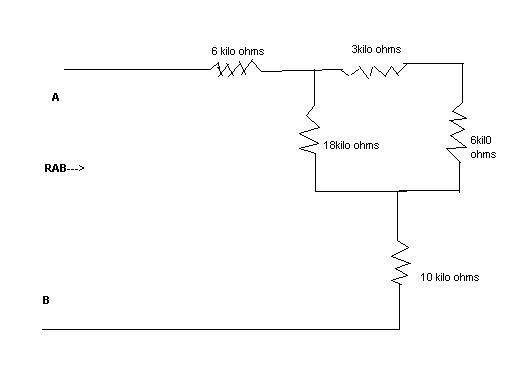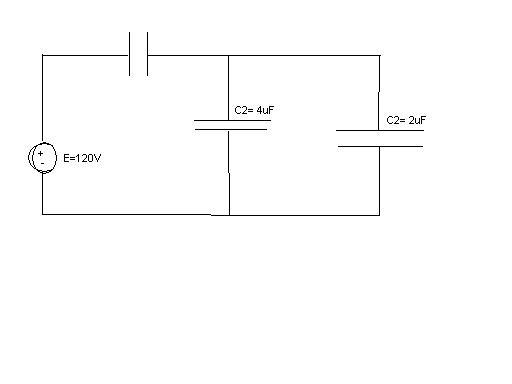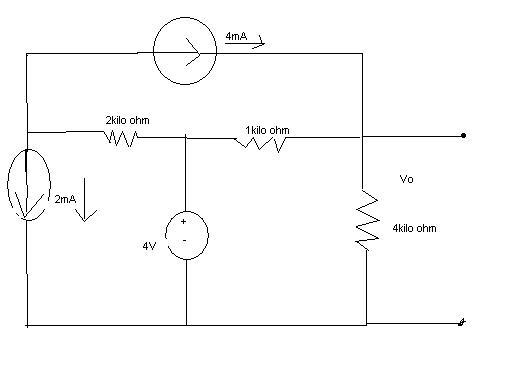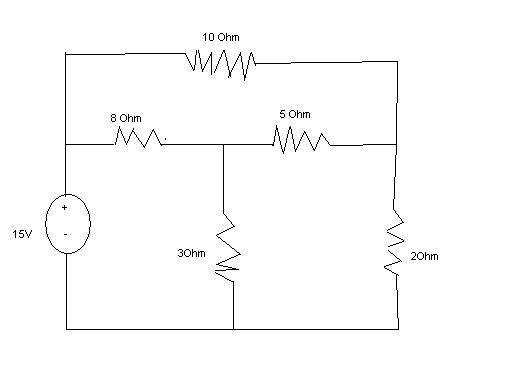ok guys, I was on a vacation, and when I came back the professor just handed out an assignment, and I have no clue about it !!!

Please please, I really need your help on this. The assignment is due on the 28th !! Please I beg you to solve this for me !

I came back late, so I only have 2 days for it, as the rest of the class had the whole week ! pleaseeeeeeeeee i really need your help!

these are the questions :

Q1) Find the equivalent resistance at terminals A-B in the circuit.------------------------------------------------------------------------

Q2) Find the voltage across and charge on each capacitor for the network below :----------------------------------------------------------------------------

Q3) State and prove the law :-

e=Ndφ/dt

--------------------------------------------------------------------------------

Q4) Find Vo in the network :-------------------------------------------------------------------------------

Q5) A factory has a 240-V supply from which the following loads are taken :

Lightning: Three hundred 150kW, four hundred 100W, and five hundred 60W lamps.

Heating : 100kW
Motors : A total of 44.76kW with an average efficiency of 75%

Misc. : Various loads taking a current of 40A

Assuming that the lightning load is on for a period of 4 hours a day, the heating for 10 hours a day, and the remaining for 2 hours a day.

Calculate the weekly consumption of the factory in Kwh when working on a 5 day week.

---------------------------------------------------------------------------

Q6) Use Mesh equations to find the current through 10 Ohm resistors.Come on, try and make an attempt.

Q1. Use the formulas for getting the total resistance of resistors in series and resistors in parallel separately.
Q2. Learn how to calculate total capacitance of capacitors in parallel
Q3. Read up on electromagnetic induction
Q4. Same principles as question 1.
Q5. Read up on Ohm's law and Coulomb's Law.
Q6. Read up on mesh analysis.

You have enough time to learn this in two days trust me.

Last edited:
Come on, try and make an attempt.

Q1. Use the formulas for getting the total resistance of resistors in series and resistors in parallel separately.
Q2. Learn how to calculate total capacitance of capacitors in parallel
Q3. Read up on electromagnetic induction
Q4. Same principles as question 1.
Q5. Read up on Ohm's law and Coulomb's Law.
Q6. Read up on mesh analysis.

You have enough time to learn this in two days trust me.

well I have solved Q no.1 and Q no.4, but I am STRUGGLING with the rest, pleaaase help :(

cristo
Staff Emeritus
well I have solved Q no.1 and Q no.4, but I am STRUGGLING with the rest, pleaaase help :(

Qno.1)
the 3 kOhm + 6 kOhm = 9 kOhm, which is in parallel with the 18 kOhm resistor. 1/R = 1/18 + 1/9. R = 6 kOhm. So, overall, that part has 6 kOhms in resistance. adding the other two resistors that are in series, 6 + 6 + 10 = 22 kOhm. the equivalent resistance is 22 kOhms.

Also Q no,3 I got help from wikipedia and ive got that done now.

So I onlyneed help with Q no.2,5 and 6.

trust me bro, I would have solved the other ones if I could but I cant. Its not that I am not investing time into studying and solving them myself, its that I dont have anymore time !

The Mesh equation question is totally out of knowledge, since he taught that while I was gona and gave this assignment.

I really need the marks on this assignment, since ive been a little off in this course.